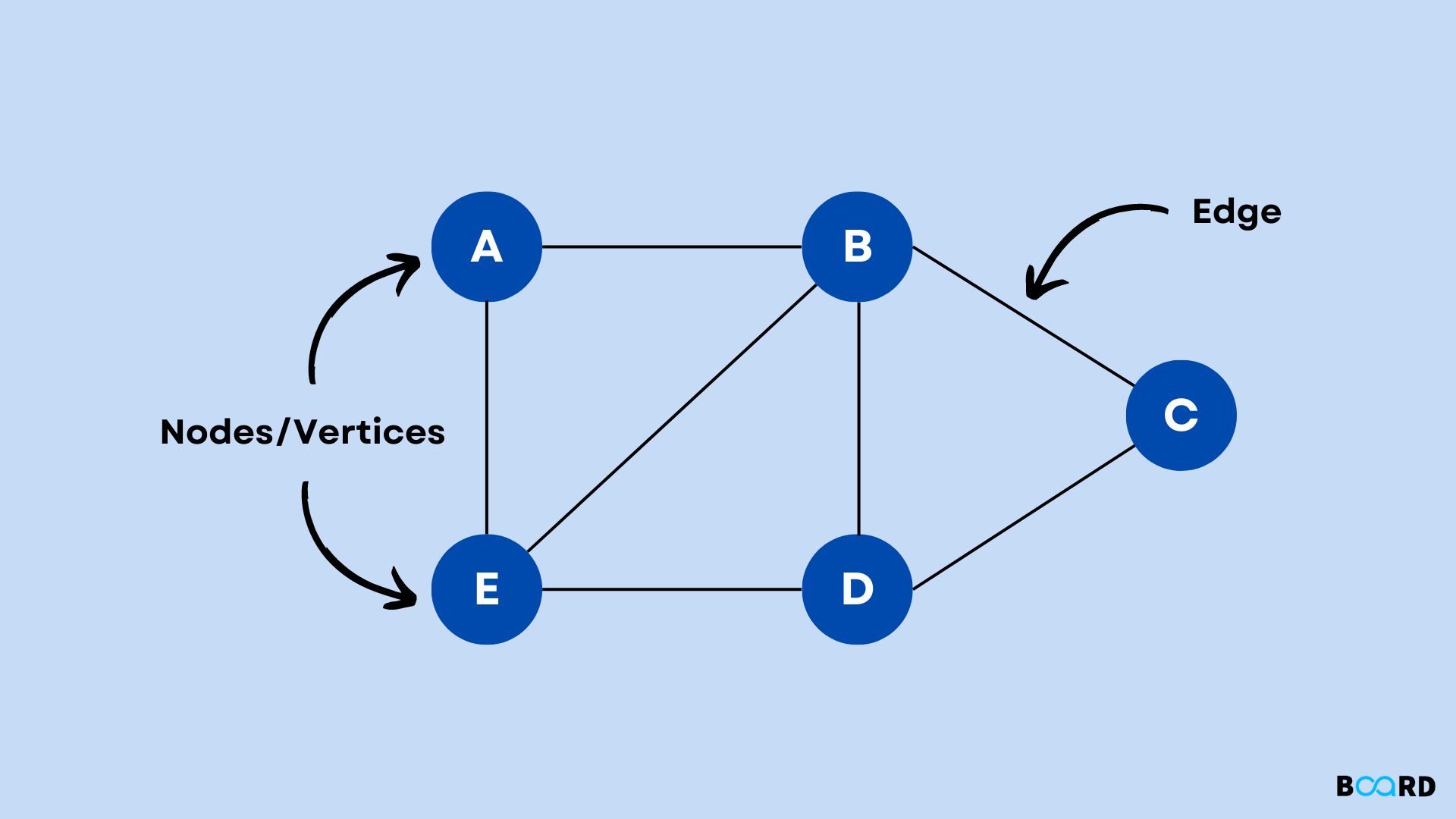# Graphs in Data Structure and AlgorithmIn this article, we will discuss graph data structure and algorithms. A graph is a non-linear data structure that consists of nodes or vertices. These vertices may or may not be connected with lines known as edges. In simple words, we can say that a graph is a collection of vertices and edges. The set of vertices is denoted by (V) and the set of edges is denoted by (E). The graph itself is denoted by G(V, E).

## Types of Graph

The graph can be classified into different categories, the most common one is a directed or undirected graph.

1. Directed graphA directed graph is a type of graph in which edges are unidirectional or they point towards a particular node to which it connects.

2. Undirected graph: An undirected graph is a type of graph in which edges don’t point to either of the attached nodes.

## Components of a Graph

The two components of a graph are the following:

• Vertex: A vertex is regarded as the fundamental unit of a graph. It is sometimes called a node. A node or vertex can be labeled or it can be unlabeled.
• Edge: The main purpose of an edge is to connect two vertices. Two nodes can be connected with an edge in any possible way. Just like a vertex, an edge can be labeled or unlabeled. An edge could carry some integer value as well known as weight.

Let us consider the following program that constructs a graph:

 // Include library#include using namespace std;// Template to support// the nodes of any data typetemplate// Graph classclass Graph{        // Unordered map to construct the edges unordered_map> edges;public:    // Add an edge void add_edge(T node1 , T node2) { edges[node1].push_back(node2); edges[node2].push_back(node1); }};int main() {        // Create an object    Graph graph;        // Add edges and construct the graph graph.add_edge(1, 2); graph.add_edge(1, 3); graph.add_edge(1, 4); graph.add_edge(3, 4);    return 0;}
write your code here: Coding Playground

The above code constructs the following graph:

## Real-life applications

The graph has many real-life applications in our day-to-day life. Some of them are listed below:

1. The graph data structure can be used to represent a network. The city can be represented by nodes and the path between cities may be represented by the edge.

2. The social media networking sites like Linkedin and Facebook can also be represented by graphs.

3.  Graph algorithm can also be used for directions in a graph. Moreover, the graph algorithms are applied to find the shortest distance between two locations.

4. Most of us know are very well aware of the sudoku game. It is a popular puzzle game with a 9x9 grid that is required to be filled using numbers from 1 to 9. We can use graph coloring to solve a sudoku game.

## Conclusion

In this article, we discussed graph data structure and algorithms. We also demonstrated how we can represent a graph programmatically. In the end, we discussed the real-life applications of graphs. We believe that you must have learned something interesting from this article